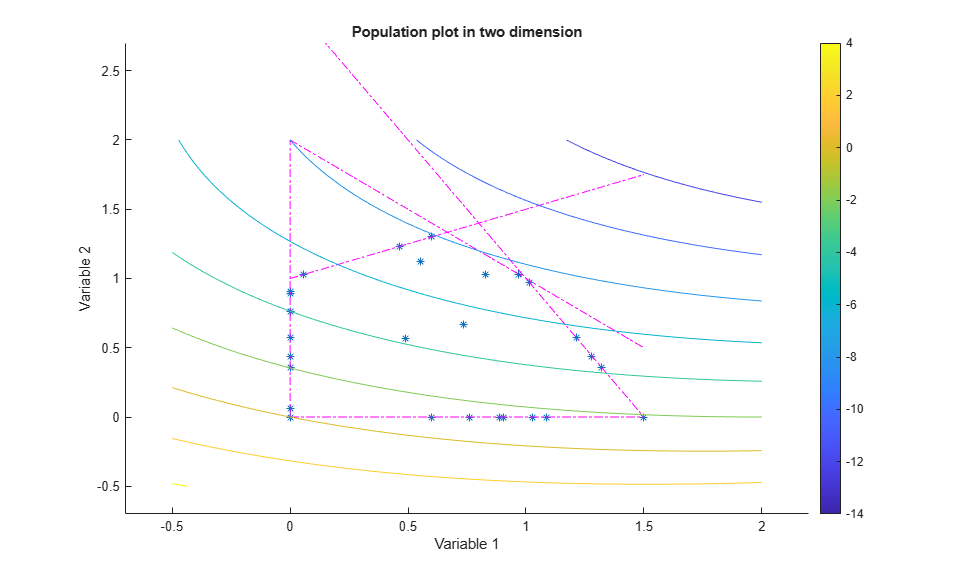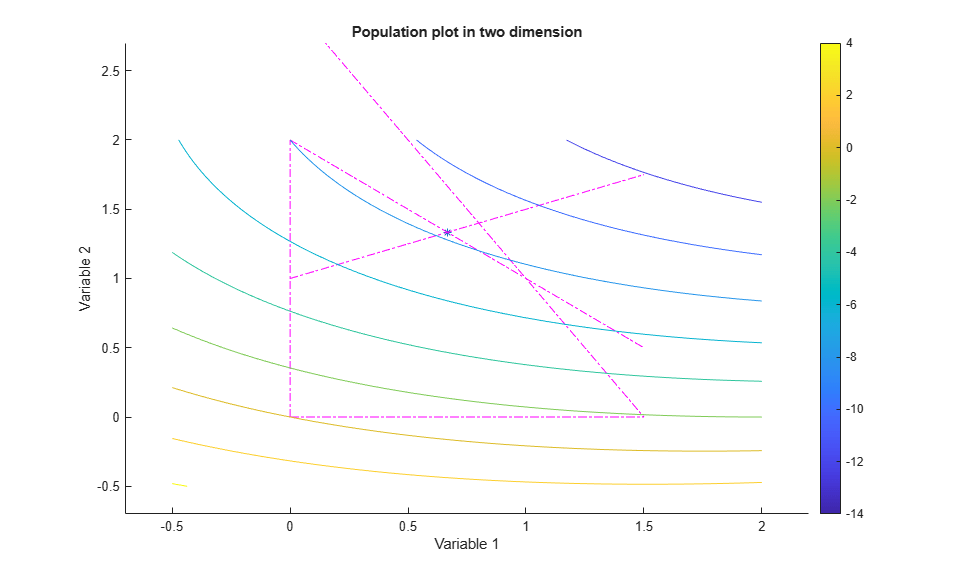Documentation

### This is machine translation

Mouseover text to see original. Click the button below to return to the English version of the page.

## Population Diversity

### Importance of Population Diversity

One of the most important factors that determines the performance of the genetic algorithm performs is the diversity of the population. If the average distance between individuals is large, the diversity is high; if the average distance is small, the diversity is low. Getting the right amount of diversity is a matter of start and error. If the diversity is too high or too low, the genetic algorithm might not perform well.

This section explains how to control diversity by setting the Initial range of the population. Setting the Amount of Mutation describes how the amount of mutation affects diversity.

This section also explains how to set the population size.

### Setting the Initial Range

By default, `ga` creates a random initial population using a creation function. You can specify the range of the vectors in the initial population in the Initial range field in Population options.

### Note

The initial range restricts the range of the points in the initial population by specifying the lower and upper bounds. Subsequent generations can contain points whose entries do not lie in the initial range. Set upper and lower bounds for all generations in the Bounds fields in the Constraints panel.

If you know approximately where the solution to a problem lies, specify the initial range so that it contains your guess for the solution. However, the genetic algorithm can find the solution even if it does not lie in the initial range, if the population has enough diversity.

The following example shows how the initial range affects the performance of the genetic algorithm. The example uses Rastrigin's function, described in Minimize Rastrigin's Function. The minimum value of the function is 0, which occurs at the origin.

To run the example, open the `ga` solver in the Optimization app by entering `optimtool('ga')` at the command line. Set the following:

• Set Fitness function to `@rastriginsfcn`.

• Set Number of variables to `2`.

• Select Best fitness in the Plot functions pane of the Options pane.

• Select Distance in the Plot functions pane.

• Set Initial range in the Population pane of the Options pane to `[1;1.1]`.

Click in Run solver and view results. Although the results of genetic algorithm computations are random, your results are similar to the following figure, with a best fitness function value of approximately 2.The upper plot, which displays the best fitness at each generation, shows little progress in lowering the fitness value. The lower plot shows the average distance between individuals at each generation, which is a good measure of the diversity of a population. For this setting of initial range, there is too little diversity for the algorithm to make progress.

To run this problem using command-line functions:

```options = optimoptions('ga','InitialPopulationRange',[1;1.1],... 'PlotFcn',{@gaplotbestf,@gaplotdistance}); x = ga(@rastriginsfcn,2,[],[],[],[],[],[],[],options)```

Next, try setting Initial range to `[1;100]` and running the algorithm. This time the results are more variable. You might obtain a plot with a best fitness value of about 7, as in the following plot. You might obtain different results.This time, the genetic algorithm makes progress, but because the average distance between individuals is so large, the best individuals are far from the optimal solution.

To run this problem using command-line functions:

```options = optimoptions('ga','InitialPopulationRange',[1;100],... 'PlotFcn',{@gaplotbestf,@gaplotdistance}); x = ga(@rastriginsfcn,2,[],[],[],[],[],[],[],options)```

Finally, set Initial range to `[1;2]` and run the genetic algorithm. Again, there is variability in the result, but you might obtain a result similar to the following figure. Run the optimization several times, and you eventually obtain a final point near `[0;0]`, with a fitness function value near `0`.The diversity in this case is better suited to the problem, so `ga` usually returns a better result than in the previous two cases.

To run this problem using command-line functions:

```options = optimoptions('ga','InitialPopulationRange',[1;2],... 'PlotFcn',{@gaplotbestf,@gaplotdistance}); x = ga(@rastriginsfcn,2,[],[],[],[],[],[],[],options)```

### Custom Plot Function and Linear Constraints in `ga`

This example shows how `@gacreationlinearfeasible`, the default creation function for linearly constrained problems, creates a population for `ga`. The population is well-dispersed, and is biased to lie on the constraint boundaries. The example uses a custom plot function.

Fitness Function

The fitness function is `lincontest6`, included with your software. This is a quadratic function of two variables:

``

Custom Plot Function

Save the following code to a file on your MATLAB® path named `gaplotshowpopulation2`.

```function state = gaplotshowpopulation2(~,state,flag,fcn) %gaplotshowpopulation2 Plots the population and linear constraints in 2-d. % STATE = gaplotshowpopulation2(OPTIONS,STATE,FLAG) plots the population % in two dimensions. % % Example: % fun = @lincontest6; % options = gaoptimset('PlotFcn',{{@gaplotshowpopulation2,fun}}); % [x,fval,exitflag] = ga(fun,2,A,b,[],[],lb,[],[],options); % This plot function works in 2-d only if size(state.Population,2) > 2 return; end if nargin < 4 fcn = []; end % Dimensions to plot dimensionsToPlot = [1 2]; switch flag % Plot initialization case 'init' pop = state.Population(:,dimensionsToPlot); plotHandle = plot(pop(:,1),pop(:,2),'*'); set(plotHandle,'Tag','gaplotshowpopulation2') title('Population plot in two dimension','interp','none') xlabelStr = sprintf('%s %s','Variable ', num2str(dimensionsToPlot(1))); ylabelStr = sprintf('%s %s','Variable ', num2str(dimensionsToPlot(2))); xlabel(xlabelStr,'interp','none'); ylabel(ylabelStr,'interp','none'); hold on; % plot the inequalities plot([0 1.5],[2 0.5],'m-.') % x1 + x2 <= 2 plot([0 1.5],[1 3.5/2],'m-.'); % -x1 + 2*x2 <= 2 plot([0 1.5],[3 0],'m-.'); % 2*x1 + x2 <= 3 % plot lower bounds plot([0 0], [0 2],'m-.'); % lb = [ 0 0]; plot([0 1.5], [0 0],'m-.'); % lb = [ 0 0]; set(gca,'xlim',[-0.7,2.2]) set(gca,'ylim',[-0.7,2.7]) axx = gcf; % Contour plot the objective function if ~isempty(fcn) range = [-0.5,2;-0.5,2]; pts = 100; span = diff(range')/(pts - 1); x = range(1,1): span(1) : range(1,2); y = range(2,1): span(2) : range(2,2); pop = zeros(pts * pts,2); values = zeros(pts,1); k = 1; for i = 1:pts for j = 1:pts pop(k,:) = [x(i),y(j)]; values(k) = fcn(pop(k,:)); k = k + 1; end end values = reshape(values,pts,pts); contour(x,y,values); colorbar end % Show the initial population ax = gca; fig = figure; copyobj(ax,fig);colorbar % Pause for three seconds to view the initial plot, then resume figure(axx) pause(3); case 'iter' pop = state.Population(:,dimensionsToPlot); plotHandle = findobj(get(gca,'Children'),'Tag','gaplotshowpopulation2'); set(plotHandle,'Xdata',pop(:,1),'Ydata',pop(:,2)); end ```

The custom plot function plots the lines representing the linear inequalities and bound constraints, plots level curves of the fitness function, and plots the population as it evolves. This plot function expects to have not only the usual inputs `(options,state,flag)`, but also a function handle to the fitness function, `@lincontest6` in this example. To generate level curves, the custom plot function needs the fitness function.

Problem Constraints

Include bounds and linear constraints.

```A = [1,1;-1,2;2,1]; b = [2;2;3]; lb = zeros(2,1); ```

Options to Include Plot Function

Set options to include the plot function when `ga` runs.

```options = optimoptions('ga','PlotFcns',... {{@gaplotshowpopulation2,@lincontest6}}); ```

Run Problem and Observe Population

The initial population, in the first plot, has many members on the linear constraint boundaries. The population is reasonably well-dispersed.

```rng default % for reproducibility [x,fval] = ga(@lincontest6,2,A,b,[],[],lb,[],[],options); ```
```Optimization terminated: average change in the fitness value less than options.FunctionTolerance. ````ga` converges quickly to a single point, the solution.

### Setting the Population Size

The Population size field in Population options determines the size of the population at each generation. Increasing the population size enables the genetic algorithm to search more points and thereby obtain a better result. However, the larger the population size, the longer the genetic algorithm takes to compute each generation.

### Note

You should set Population size to be at least the value of Number of variables, so that the individuals in each population span the space being searched.

You can experiment with different settings for Population size that return good results without taking a prohibitive amount of time to run.

Watch now